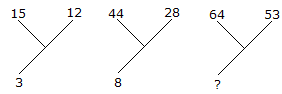# Verbal Reasoning - Character Puzzles - Discussion

### Discussion :: Character Puzzles - Character Puzzles 1 (Q.No.28)

28.

Which one will replace the question mark ?[A]. 30 [B]. 13 [C]. 70 [D]. 118

Explanation:

(15 + 12)/9 = 3

and (44 + 28)/9 = 8

Therefore, (64 + 53)/9 = 13.

 Vidhya said: (Jul 9, 2014) Hai, I solve this problem in another way. But, I don't know this is correct or not. 3+5 = 8. 8+5 = 13. I want this answer is correct or not?

 Srini said: (Jul 1, 2016) @Vidhya: Your method is wrong because try to use all terms given in the question.

 Hitakshi said: (Oct 21, 2016) From where this 9 comes?

 Ashwini said: (May 22, 2018) How do we find upward value total /lower value? Please explain.

 Kiranmai said: (Sep 23, 2020) How come 9 here?

 Swathi said: (Oct 15, 2020) How the number 9 comes? Explain it.